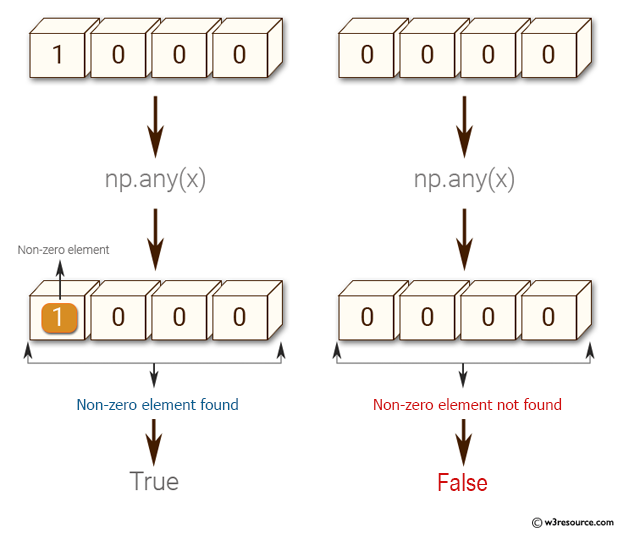﻿ NumPy: Test if any of the elements of a given array is non-zero - w3resource

# NumPy: Test if any of the elements of a given array is non-zero

## NumPy: Basic Exercise-4 with Solution

Write a NumPy program to test if any of the elements of a given array is non-zero.

Sample Solution :

Python Code :

``````import numpy as np
x = np.array([1, 0, 0, 0])
print("Original array:")
print(x)
print("Test if any of the elements of a given array is non-zero:")
print(np.any(x))
x = np.array([0, 0, 0, 0])
print("Original array:")
print(x)
print("Test if any of the elements of a given array is non-zero:")
print(np.any(x))
``````

Sample Output:

```Original array:
[1 0 0 0]
Test if any of the elements of a given array is non-zero:
True
Original array:
[0 0 0 0]
Test if any of the elements of a given array is non-zero:
False
```

Pictorial Presentation:Python Code Editor:

Have another way to solve this solution? Contribute your code (and comments) through Disqus.

What is the difficulty level of this exercise?

﻿

Inviting useful, relevant, well-written and unique guest posts